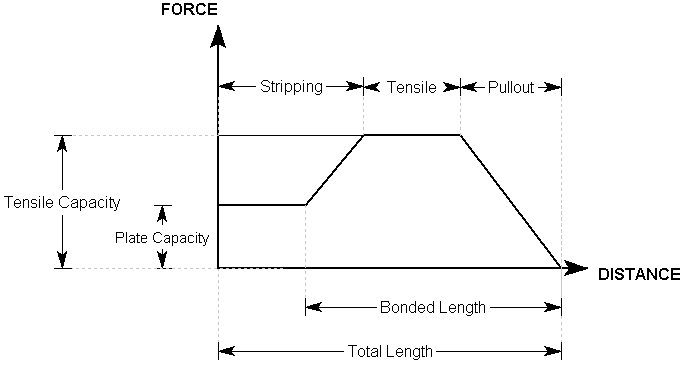Expert Modelling: Hands-on help from Rocscience Engineers. Register Here

# Bolt Force Diagrams

A Bolt Force Diagram, as defined in SWedge, represents the available tensile force which a bolt can apply to a wedge, at any point along the length of the bolt. That is, if a wedge plane intersects a bolt at a certain location along its length, what is the maximum tensile support force that the bolt can apply to the wedge. This assumes that the bolt is mobilized in pure tension.

• For Mechanically Anchored bolts, the Force Diagram is simply a horizontal line, since a constant support force will be applied, regardless of where a wedge plane intersects the bolt.
• For Grouted Dowel, Cable, Split Set or Swellex bolts, the Force diagram is determined by considering each possible tensile failure mode along the length of the support (Tensile, Pullout and Stripping, if applicable). The Failure Mode which generates the MINIMUM force determines the support force which can be generated, at each point along the support.
• For User Defined Support, the Force Diagram is defined explicitly by the user, tensile properties (e.g. tensile strength, plate capacity, bond strength) are not entered.

For example, a possible Bolt Force Diagram for a grouted dowel, with a Bond Length less than 100%, and a plate capacity which is less than the tensile capacity, is illustrated below.Bolt Force Diagram for Grouted Dowel

For more examples of typical Bolt Force Diagrams, see the following topics:

Mechanically Anchored Bolt

Grouted Dowel

Cable Bolt

Split Set / Swellex

User Defined

In addition to the Bolt Force Diagram, there are two other factors which ultimately determine the support force which can be supplied by a bolt in SWedge - Bolt Orientation Efficiency and Shear Strength. See these topics for more information.

For an overview of the bolt support implementation in SWedge, see the Bolt Support Force topic. This discusses all of the parameters which affect the bolt support capacity, and summarizes all possible cases.

## Interpretation of Bolt Force Diagrams

The user should appreciate that a Bolt Force Diagram, as implemented in SWedge, does not necessarily represent the true state of stress or loading mobilized by a given bolt. The Force diagram simply represents the maximum available tensile force, which a given bolt can theoretically apply to a wedge, at any point along its length. This is based on the assumptions described for each bolt type in SWedge.

Remember that the SWedge analysis is a limit equilibrium analysis. Displacements and strains are not considered in such an analysis. In order to realistically model the interaction of support and excavation, and to calculate actual strains and loading mobilized within the support, more sophisticated numerical analysis methods are required, such as finite element or finite difference methods.

The modeling of support in SWedge is a simple but useful method, of accounting for the effect of the support system on the wedge, in a limit equilibrium analysis. It is not intended to model the effect of the wedge on the support system, and the Bolt Force Diagrams should not be interpreted in this way.